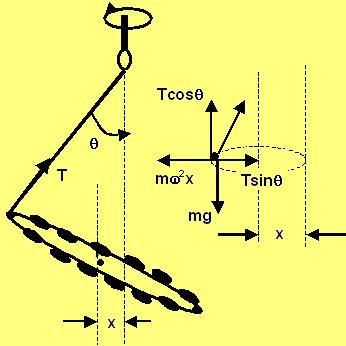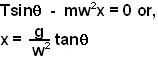## Friday, May 9, 2008

### Irodov Problem 1.145No matter how the different parts of the chain move, the dynamics of the center of mass of the chain act as if it were a point particle with the entire mass of the chain m concentrated at the point. The sum of all the forces acting on different parts of the chain then, becomes the force acting on the center of mass. We can conveniently use this fact about the center of mass to solve the problem.

Suppose that the CG is x units off from the axis of rotation. As seen by an observer in the rotating reference frame, there are three forces acting on the center of mass, i) tension in the string T, ii) the force of gravity mg and iii) the centripetal force. In the rotating reference frame, the CG is not accelerating .

In the vertical direction we have,In the horizontal direction we have,